# Limit cycle

(diff) ← Older revision | Latest revision (diff) | Newer revision → (diff)

A closed trajectory in the phase space of an autonomous system of ordinary differential equations that is an- or-limit set (cf. Limit set of a trajectory) of at least one other trajectory of this system. A limit cycle is called orbit stable, or stable, if for anythere is a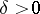such that all trajectories starting in a-neighbourhood of it for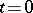do not leave its-neighbourhood for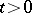(cf. Orbit stability). A limit cycle corresponds to a periodic solution of the system, differing from a constant. In order for a periodic solution to correspond to a stable limit cycle it is sufficient that the moduli of all its multipliers, except one, be less than one (cf. Characteristic exponent; Andronov–Witt theorem). From the physical point of view, a limit cycle corresponds to periodic behaviour, or an auto-oscillation, of the system (cf. ).

Suppose that an autonomous system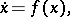(*)

defined in a region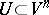, where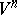is a differentiable manifold, e.g.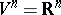, has a closed trajectory. Draw the hyperplaneintersectingtransversally at a point. Then every trajectory of the system starting forat a point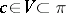, witha sufficiently small neighbourhood of, intersectsagain, at a point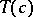, asincreases. The diffeomorphismhas fixed pointand is called the Poincaré return map. Its properties determine the behaviour of trajectories of the system in a neighbourhood of. A limit cycle, as distinct from an arbitrary closed trajectory, always determines a Poincaré return map that is not the identity. Ifis a saddle point of the diffeomorphism, then the limit cycleis said to be of saddle type. A system having a limit cycle of saddle type can have homoclinic curves, i.e. trajectories for which the limit cycle is both the- and the-limit set.

In the case of a two-dimensional system (*)one takes a straight line forand considers the function,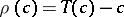, which is called the Poincaré return function. The multiplicity of the zero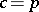ofis called the multiplicity of the limit cycle. A limit cycle of even multiplicity is called semi-stable. The limit cycles, together with the rest points and the separatrices (cf. Separatrix), determine the qualitative picture of the behaviour of the other trajectories (cf. Poincaré–Bendixson theory, as well as , ). In the case of an analytic functionthe limit cycles belong to one of the following three types: 1) stable; 2) unstable, i.e. stable if the direction ofis reversed; or 3) semi-stable. E.g., the system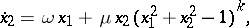where,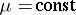,, has for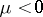(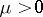) andodd a stable (unstable) limit cycle of multiplicity, while foreven it has a limit cycle of multiplicity. In all cases the limit cycle is the circle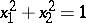, i.e. the trajectory of the solution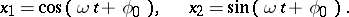If the system (*) is given on a simply-connected domain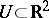, then a limit cycle encircles at least one rest point of the system.

In order to find limit cycles of second-order systems one uses methods based on the following fact: If a vector fieldis directed inwards (outwards) an annular domainand ifdoes not contain rest points, then there is at least one stable (unstable) limit cycle in. The choice ofis based on physical considerations or results from analytic or numerical computations.

How to Cite This Entry:
Limit cycle. Encyclopedia of Mathematics. URL: http://encyclopediaofmath.org/index.php?title=Limit_cycle&oldid=15601
This article was adapted from an original article by L.A. Cherkas (originator), which appeared in Encyclopedia of Mathematics - ISBN 1402006098. See original article